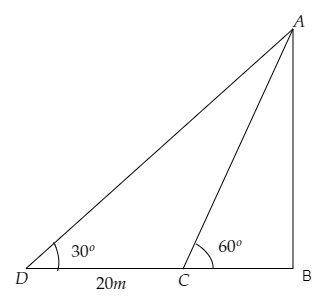# A T.V. Tower stands vertically on a bank of a river. From a point on the other bank directly opposite the tower, the angle of elevation of the top of the tower is $60^{\circ}$. From a point $20 \mathrm{~m}$ away this point on the same bank, the angle of elevation of the top of the tower is $30^{\circ}$. Find the height of the tower and the width of the river.

Given:

A T.V. Tower stands vertically on a bank of a river. From a point on the other bank directly opposite the tower, the angle of elevation of the top of the tower is $60^{\circ}$. From a point $20 \mathrm{~m}$ away this point on the same bank, the angle of elevation of the top of the tower is $30^{\circ}$.

To do:

We have to find the height of the tower and the width of the river.

Solution:Let $AB$ be the height of the tower and $BC$ be the width of the river.

Let point $C$ be the point of observation on the other bank and point $D$ be $20 \mathrm{~m}$ away from point $C$ on the same bank.

From the figure,

$\mathrm{CD}=20 \mathrm{~m}, \angle \mathrm{ADB}=30^{\circ}, \angle \mathrm{ACB}=60^{\circ}$

Let the height of the tower be $\mathrm{AB}=h \mathrm{~m}$ and the width of the river be $\mathrm{BC}=x \mathrm{~m}$.

This implies,

$\mathrm{DB}=20+x \mathrm{~m}$

We know that,

$\tan \theta=\frac{\text { Opposite }}{\text { Adjacent }}$

$=\frac{\text { AB }}{BC}$

$\Rightarrow \tan 60^{\circ}=\frac{h}{x}$

$\Rightarrow \sqrt3=\frac{h}{x}$

$\Rightarrow x(\sqrt3)=h \mathrm{~m}$

$\Rightarrow h=x\sqrt3 \mathrm{~m}$.........(i)

Similarly,

$\tan \theta=\frac{\text { Opposite }}{\text { Adjacent }}$

$=\frac{\text { AB }}{DB}$

$\Rightarrow \tan 30^{\circ}=\frac{h}{x+20}$

$\Rightarrow \frac{1}{\sqrt3}=\frac{x\sqrt3}{x+20}$              [From (i)]

$\Rightarrow x+20=x\sqrt3(\sqrt3) \mathrm{~m}$

$\Rightarrow 3x-x=20 \mathrm{~m}$

$\Rightarrow x=\frac{20}{2} \mathrm{~m}$

$\Rightarrow x=10 \mathrm{~m}$

$\Rightarrow h=10\sqrt3 \mathrm{~m}$

Therefore, the height of the tower is $10\sqrt3 \mathrm{~m}$ and the width of the river is $10 \mathrm{~m}$.

Updated on: 10-Oct-2022

15 Views